# Primary 6 PSLE Practice #5

Time elapsed:
Q1. Find the value of (260 - 80 + 120) ÷ (10 - 4).     [1 mark]
Q2. The pie chart shows how Yvette spent her money. How much did Yvette spend on clothes?     [1 mark]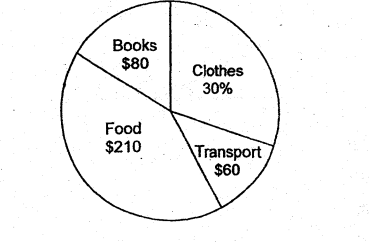Q3. Which of the following is the same as 6.05 km?     [1 mark]
Q4. Which of the following numbers is the largest?     [1 mark]
Q5. Round off 72 590 to the nearest hundred.     [1 mark]
Q6. The length of a double-decker bus in Singapore is about     [1 mark]
Q7. Which of the following is not a symmetric figure?     [2 marks]
Q8. Which of the following nets can be folded to form a cube?     [1 mark]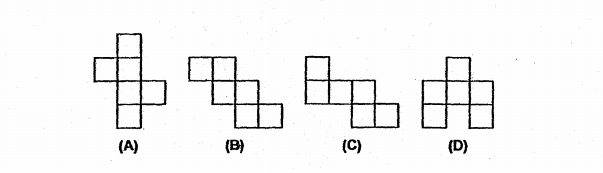Q9. What is the digit in the tenths place in the sum of 44.2 and 0.81?     [1 mark]
Q10. Round \$89 425 to the nearest \$1000.     [1 mark]
Q11. Grace is p years old. Her mother is 3 times as old as she. How old will her mother be in 4 years time?     [1 mark]
Q12. At 09 00, a lorry left Town X for Town Y travelling at a speed of 70 km/h. At the same time, a car left Town Y for Town X travelling at a speed of 90 km/h. The distance between Town X and Town Y is 480 km. At what time did the lorry and car pass each other?     [2 marks]
Q13. What is the value of (8m + 6)/6 when m = 9?     [1 mark]
Q14. In the diagram below, not drawn to scale, ACD is an equilateral triangle and BCD is a straight line. Find ∠y.     [1 mark]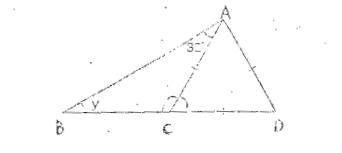Q15. Which one of the following is a net of a cube?     [1 mark]
Q16. Mark uses the four letters, C, A, R, E, to form a pattern. The first 16 letters are shown below. Which letter is in the 59th position?     [2 marks]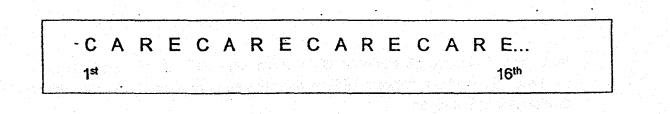Q17. Which of the following numbers is the smallest?     [1 mark]
Q18. Read the riddle below. Which one of the following is the correct shape?     [1 mark]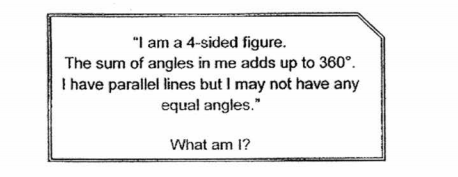Q19. The figure below shows a rhombus. Which of the following is true?     [1 mark]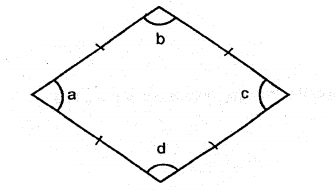Q20. Round off 41 856 to the nearest thousands.     [1 mark]